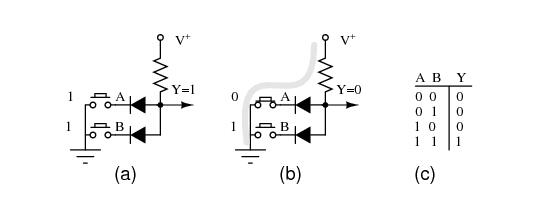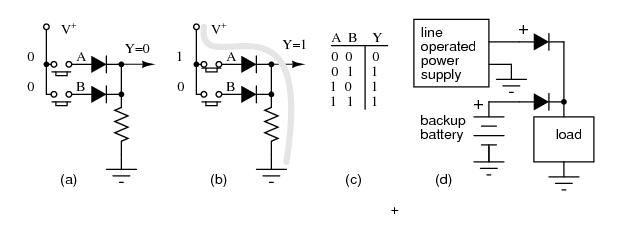# Diode Switching Circuits

Diodes can perform switching and digital logic operations. Forward and reverse bias switch a diode between the low and high impedance states, respectively. Thus, it serves as a switch.

Logic

Diodes can perform digital logic functions: AND, and OR. Diode logic was used in early digital computers. It only finds limited application today. Sometimes it is convenient to fashion a single logic gate from a few diodes.Diode AND Gate

An AND gate is shown in Figure above. Logic gates have inputs and an output (Y) which is a function of the inputs. The inputs to the gate are high (logic 1), say 10 V, or low, 0 V (logic 0). In the figure, the logic levels are generated by switches. If a switch is up, the input is effectively high (1). If the switch is down, it connects a diode cathode to ground, which is low (0). The output depends on the combination of inputs at A and B. The inputs and output are customarily recorded in a “truth table” at (c) to describe the logic of a gate. At (a) all inputs are high (1). This is recorded in the last line of the truth table at (c). The output, Y, is high (1) due to the V+ on the top of the resistor. It is unaffected by open switches. At (b) switch A pulls the cathode of the connected diode low, pulling output Y low (0.7 V). This is recorded in the third line of the truth table. The second line of the truth table describes the output with the switches reversed from (b). Switch B pulls the diode and output low. The first line of the truth table recordes the Output=0 for both input low (0). The truth table describes a logical AND function. Summary: both inputs A and B high yields a high (1) out.

A two input OR gate composed of a pair of diodes is shown in Figure below. If both inputs are logic low at (a) as simulated by both switches “downward,” the output Y is pulled low by the resistor. This logic zero is recorded in the first line of the truth table at (c). If one of the inputs is high as at (b), or the other input is high, or both inputs high, the diode(s) conduct(s), pulling the output Y high. These results are reordered in the second through fourth lines of the truth table. Summary: any input “high” is a high out at Y.OR gate: (a) First line, truth table (TT). (b) Third line TT. (d) Logical OR of power line supply and back-up battery.

A backup battery may be OR-wired with a line operated DC power supply in Figure above (d) to power a load, even during a power failure. With AC power present, the line supply powers the load, assuming that it is a higher voltage than the battery. In the event of a power failure, the line supply voltage drops to 0 V; the battery powers the load. The diodes must be in series with the power sources to prevent a failed line supply from draining the battery, and to prevent it from over charging the battery when line power is available. Does your PC computer retain its BIOS setting when powered off? Does your VCR (video cassette recorder) retain the clock setting after a power failure? (PC Yes, old VCR no, new VCR yes.)

Get the latest price? We'll respond as soon as possible(within 12 hours)